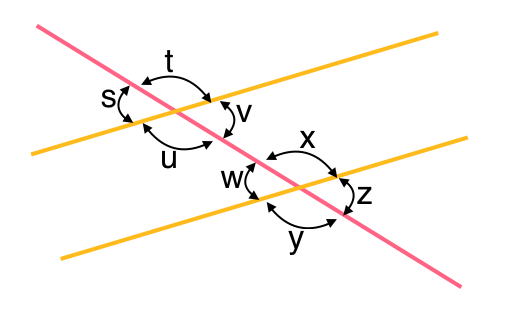Angle Relationships
starstarstarstarstarstarstarstarstarstar
by Hannah Ryan| 8 Questions

# Use the worksheet below to explore angle relationships from parallel lines cut by a transversal.

1
1
If <ABC equals 45º, what is the angle of <CBE ?
45º
50º
145º
135º

# Use the image below for Questions 1, 2, & 3.2
1

# #1. Which of the following pairs of angles are Corresponding Angles?

Select all that apply.

i & m
j & n
k & o
l & p
3
1

# #2. Which of the following pairs of angles are Alternate Exterior Angles?

Select all that apply.
angles i & p
angles j & p
angles i & o
angles j & o
4
1

# #3. Which of the following pairs of angles are Consecutive Interior Angles?

Select all that apply.

angles k & n
angles k & m
angles l & m
angles l & n

# Use the image below for Questions 4 & 5.5
1

# #4. Which of the following pairs of angles are Alternate Interior Angles?

Select all that apply.

angles u & x
angles u & w
angles v & w
angles v & x
6
1

# #5. Which of the following pairs of angles are Vertically Opposite Angles?

Select all that apply.

s & v
t & u
y & z
u & v

# Go to the website below to play a game that helps you to recognize vertically opposite, corresponding, and alternate angles formed by parallel lines and a transversal.

http://www.learnalberta.ca/content/mejhm/index.html?l=0&ID1=AB.MATH.JR.SHAP&ID2=AB.MATH.JR.SHAP.ANG&lesson=html/object_interactives/parallel_lines/use_it.html

# Drag the icons for the given building to the appropriate places.

If you need to reset your buildings, click the double-arrow icon in the upper right corner.

• The house and grocery store are alternate interior angles.
• The gas station and shopping center are alternate exterior angles.
• The grocery store and town hall are a consecutive (same side) interior angles.
• The house and park are corresponding angles.
• The town hall and gas station are vertical angles.
• The grocery store and restaurant are alternate interior angles.
• The park and barber shop are consecutive (same side) exterior angles.
• The park and the church are alternate-exterior angles.
• The church and the school are corresponding angles.
• The post office and shopping center are linear pair.
7
1
#6. If the house and the park are corresponding angles and the house has an angle of 52º, what is the angle of the park?
8
1
#8. If the town hall and gas station are vertical angles and the town hall has an angle of 60º, what is the angle of the gas station?